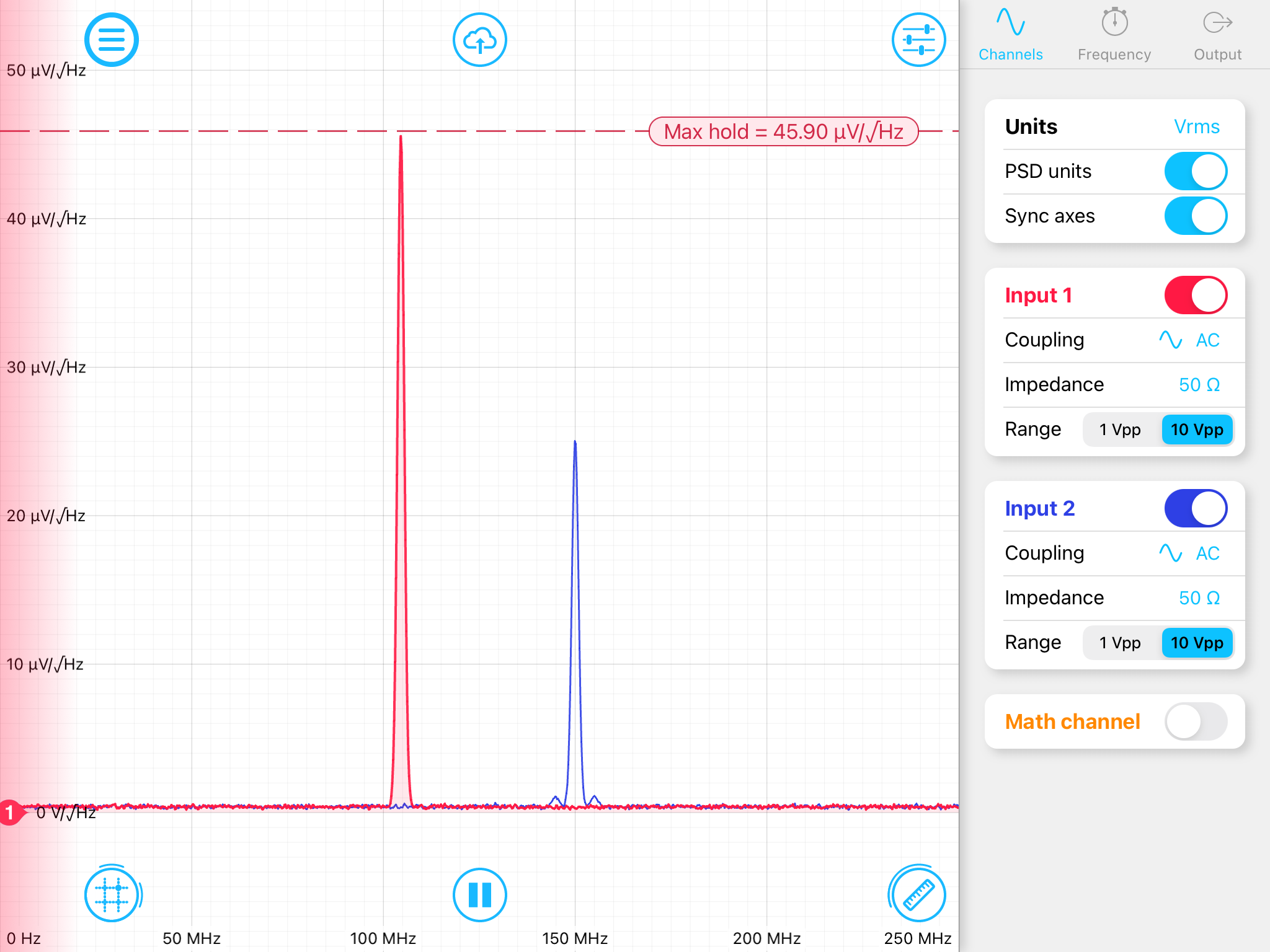# Spectrum Analyzer units of measure

Converting from dBm/Hz to V/√Hz

The Moku:Lab Spectrum Analyzer can display the spectrum amplitude in various units (dBm, Vrms, Vpp and dBV).  Additionally,  you can select corresponding power spectral density (PSD) units (dBm/Hz, Vrms/ Hz, Vpp/√Hz and dBV/ Hz).  It is worth noting that the Resolution Band Width (RBW) setting only affects the measurement in PSD units.

Below is an example from the Moku:Lab demo mode,  the channel 1 peak is shown at -73.75 dBm/Hz.This can be converted to Vrms/Hz by relationship P [mW/Hz] = 10^ (P [dBm/Hz] / 10)

So in this case, P = 4.22 x 10 -8  mW/Hz

Also,  V= √( P x R) and that here 50 ohms is the conventional nominal impedance.

Thus the spectral peak in volts, allowing for conversion from mW to W, is

V = √( 50 x 4.22 x 10 -11)

V = 45.9 μV/Hz

In this second screenshot of the Moku:Lab Spectrum Analyzer demo mode,  the units have been switch to uV/Hz.